What do you know about indices and roots? Find out in this quiz.

# Indices and Roots

This Math quiz is called 'Indices and Roots' and it has been written by teachers to help you if you are studying the subject at middle school. Playing educational quizzes is a fabulous way to learn if you are in the 6th, 7th or 8th grade - aged 11 to 14.

It costs only \$12.50 per month to play this quiz and over 3,500 others that help you with your school work. You can subscribe on the page at Join Us

Using INDICES, or powers, is a shorthand way of showing that a number (or a variable) has been multiplied by itself. Taking the root of a number is this process in reverse. Take this middle school Math quiz to see how well you can cope with all the little rules!

You should know about the idea of multiplying a number by itself, or squaring it. The reverse process, taking the square root, involves finding a number that, when multiplied by itself, gives you the number you started with. Mathematicians developed the notation to use a small, raised power as a shorthand way of writing out repeated multiplication. This has been developed to include negative and fractional indices, and you should be familiar with all of them.

Learn the laws of indices to help you perform calculations involving roots and powers, and remember that ‘anything to the power of zero is always equal to 1’.

Standard form, also known as scientific notation, is a useful way of writing very large or very small numbers. Part of a number written in standard form includes 10 to the power of something. This works because big numbers, like 1,000,000 (1 million), can be written as 106. We might not be able to visualize really big numbers, but standard form at least allows us to perform calculations without getting caught up in a pile of zeroes!

1.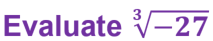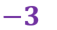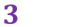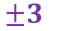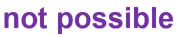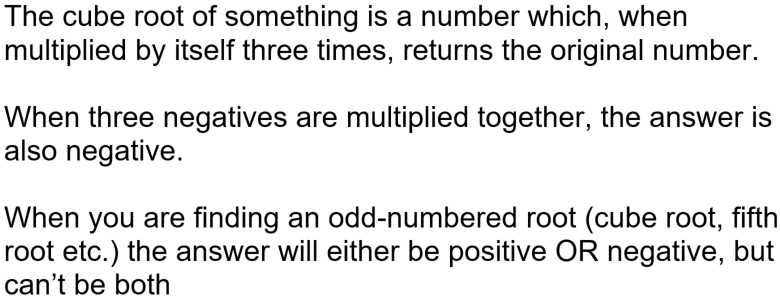2.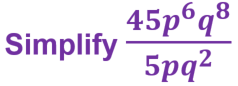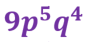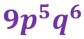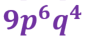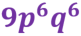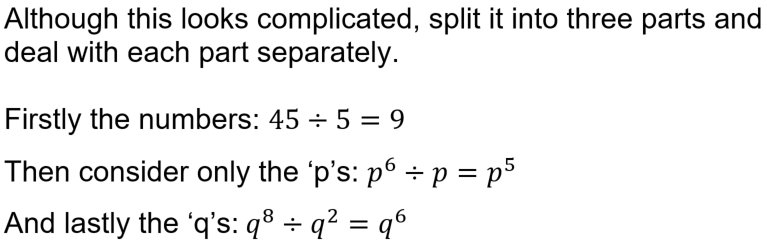3.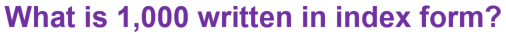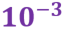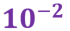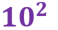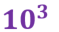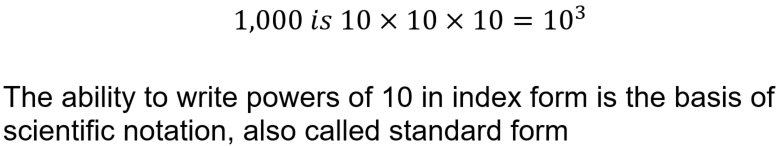4.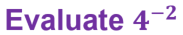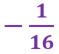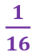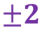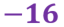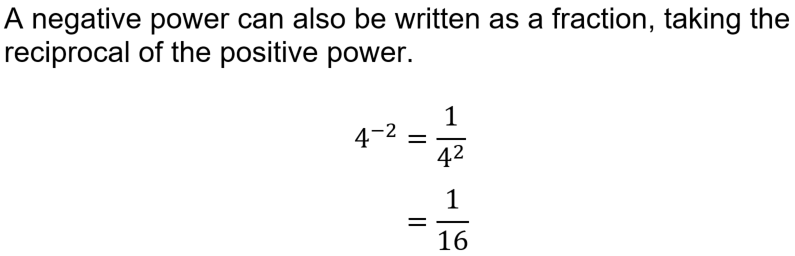5.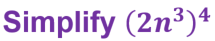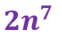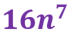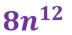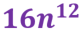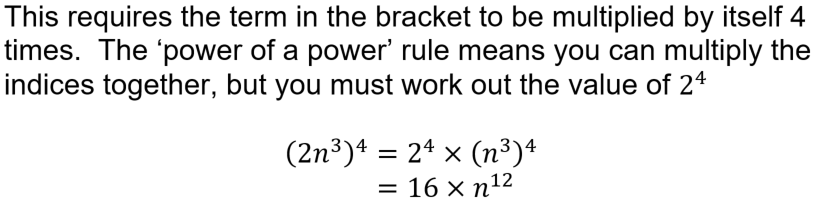6.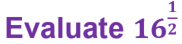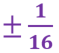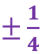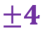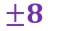7.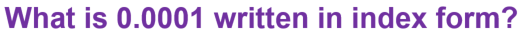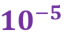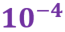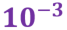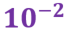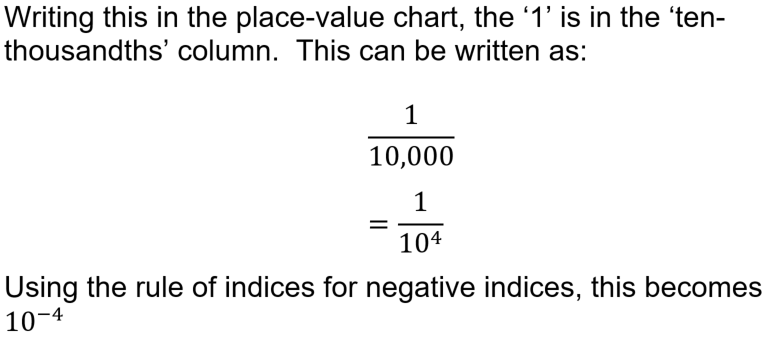8.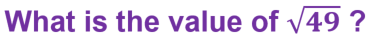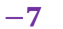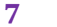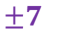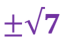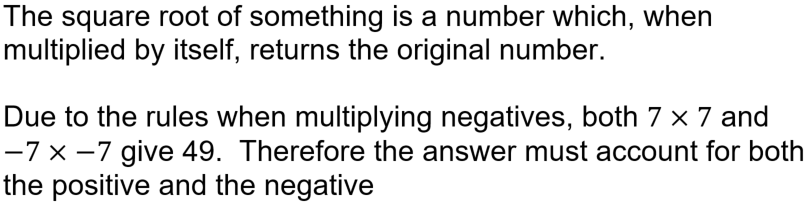9.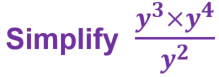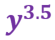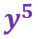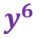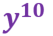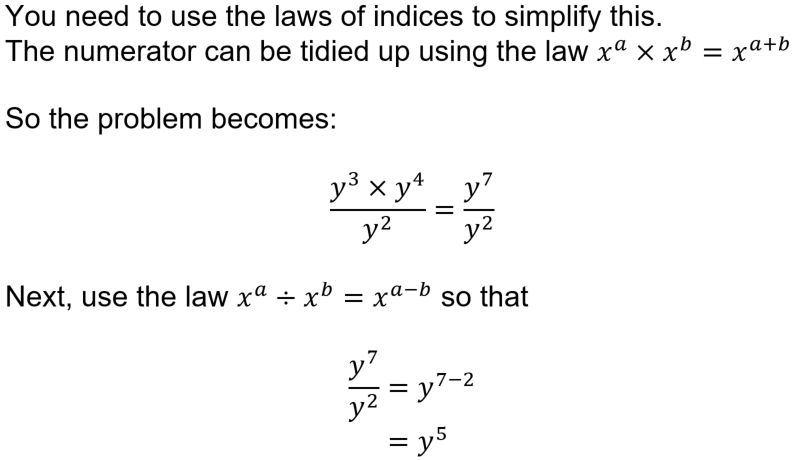10.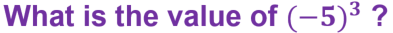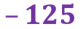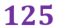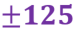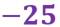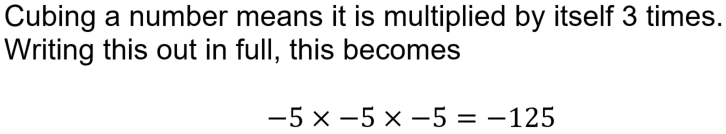Author:  Sally Thompson